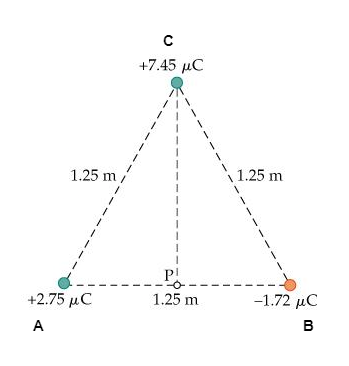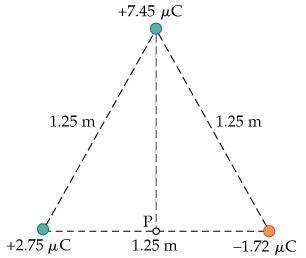# Problem: Part A. Find the electric potential at point P in the figure. VP =________VPart B. Suppose the three charges shown in the figure are held in place. A fourth charge, with a charge of +7.00 µC and a mass of 4.80 g, is released from rest at point P. What is the speed of the fourth charge when it has moved infinitely far away from the other three charges? v =________m/s

###### FREE Expert Solution

Electric potential:

$\overline{){\mathbit{V}}{\mathbf{=}}\frac{\mathbf{k}\mathbf{q}}{\mathbf{r}}}$

Electric potential energy:

$\overline{){\mathbf{U}}{\mathbf{=}}{\mathbf{q}}{\mathbf{V}}}$

Label the vertices of the triangle as follows:98% (207 ratings)###### Problem Details

Part A. Find the electric potential at point P in the figure. VP =________V

Part B. Suppose the three charges shown in the figure are held in place. A fourth charge, with a charge of +7.00 µC and a mass of 4.80 g, is released from rest at point P. What is the speed of the fourth charge when it has moved infinitely far away from the other three charges? v =________m/s